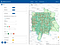# Mapping statistics between different spatial hierarchies

Today’s recipe discusses the following problem: you have some geospatial statistics collected for one set of spatial hierarchy, but you need to do some data analysis using another hierarchy. Say we have very detailed US census statistics, collected for census tracts, but we need statistics for zip codes, and we don’t have any.

Now a huge disclaimer: you should NOT use zip codes for your geospatial analysis, they are bad for that purpose. See this excellent article in Carto blog that demonstrates well why this is bad:
https://carto.com/blog/zip-codes-spatial-analysis/

But well, sometimes you need to do something even if you should not. Or rather let’s say we’ll use zip codes as convenient demonstrations, but you promise to use it for something better, maybe map to river basin areas?

So for today we want to count people and find average incomes in each zip code, but we only have census data. Note that there is no common hierarchy between postal codes and census tracts. A zip code can contain several tracts, or vice versa, or they may partially overlap.

Let’s see them in BigQuery GeoViz. It does not support multiple layers, so I’ll use the following `UNION ALL` query to visualize them together in GeoViz, adding `census` column to be able to distinguish them:

`select * from (  select     1 as census, tract_geom geom   from     `bigquery-public-data.geo_census_tracts.census_tracts_washington`  union all  select     0 as census, zip_code_geom geom   from     `bigquery-public-data.geo_us_boundaries.zip_codes`)where st_dwithin(geom, st_geogpoint(-122.191667, 47.685833), 5000)`Census tracts and zip code polygons

With the added column, we can draw zip code boundaries green, and tract boundaries red to distinguish them. And we see they are completely independent polygons.

What can we do? A simple idea is to split census tracts on zip code boundaries. We then pretend each census tract is uniform, so if 40% of tract surface area is in a zip code A, we assign 40% of the population to that zip code.

How can we compute average incomes? We cannot assign 40% of average to a zip code, like we do with people, this makes no sense. But we can assign 40% of total tract’s income, computed as `average * population` to a zip code! Note this only works with averages, but we cannot use this simple trick with medians — they cannot be mapped this simple way.

OK, let’s start coding. First, we need to find all pairwise intersections between tracts and zip codes, and for each pair compute tract’s percentage of area that we assign to zip code it intersects.

`CREATE OR REPLACE TABLE     demo.zip_tract_join ASWITH zip_tract_join AS (  SELECT     zips.zip_code,     zips.functional_status as zip_functional_status,    tracts.tract_ce,     tracts.geo_id as tract_geo_id,     tracts.functional_status as tract_functional_status,    ST_Area(ST_Intersection(tracts.tract_geom, zips.zip_code_geom))        / ST_Area(tracts.tract_geom) as tract_pct_in_zip_code  FROM      `bigquery-public-data.geo_census_tracts.us_census_tracts_national` tracts,    `bigquery-public-data.geo_us_boundaries.zip_codes` zips  WHERE     ST_Intersects(tracts.tract_geom, zips.zip_code_geom))SELECT * FROM zip_tract_join WHERE tract_pct_in_zip_code > 0;`

This creates a table `zip_tract_join` with all non-empty intersections, where `tract_pct_in_zip_code` is share of the tract that belongs to particular intersection, computed as area of intersection divided by total tract area. BTW, this query took less than 30 seconds for the whole US!

Next, remember that we need additive values, like total income, rather than averages that are hard to work with. We’ll use this query:

`SELECT geo_id, total_pop,        total_pop * income_per_capita as total_income FROM `bigquery-public-data.census_bureau_acs.censustract_2017_5yr``

Then we join this with `demo.zip_tract_join` table created in previous step, aggregate by zip code, and convert back to averages. Whole query is:

`CREATE OR REPLACE TABLE demo.zip_pop_income ASWITH census_totals AS (  -- convert averages to additive totals  SELECT     geo_id, total_pop,     total_pop * income_per_capita AS total_income   FROM     `bigquery-public-data.census_bureau_acs.censustract_2017_5yr` ),joined AS (   -- join with precomputed census/zip pairs,  -- compute zip's share of tract  SELECT     zip_code,     total_pop * tract_pct_in_zip_code    AS zip_pop,    total_income * tract_pct_in_zip_code AS zip_income  FROM census_totals c  JOIN demo.zip_tract_join ztj  ON c.geo_id = ztj.tract_geo_id),sums AS (   -- aggregate all "pieces" of zip code  SELECT    zip_code,     SUM(zip_pop) AS zip_pop,    SUM(zip_income) AS zip_total_inc  FROM joined   GROUP BY zip_code)SELECT   zip_code, zip_pop,   -- convert to averages  zip_total_inc/zip_pop AS income_per_capitaFROM sums`

We got a zip code statistics table `zip_pop_income` for the whole U.S. It does not contain zip geometry information, only statistics. If we need a specific zip code, we can query this table alone. For area query, wee can join it with zip boundaries table:

`with zipcodes_within_distance as (SELECT   zip_code, zip_code_geomFROM   `bigquery-public-data.geo_us_boundaries.zip_codes`WHERE  ST_DWithin(      ST_GeogPoint(-122.191667, 47.685833),      zip_code_geom,      1609 * 5))select   zip_code_geom, stats.*from   zipcodes_within_distance areajoin   demo.zip_pop_income statson area.zip_code = stats.zip_code`

For sanity check, let’s compare this with direct query of original census stats:

`SELECT   income_per_capita, tract_geomFROM   `bigquery-public-data.geo_census_tracts.census_tracts_washington` tJOIN  `bigquery-public-data.census_bureau_acs.censustract_2017_5yr` sON  t.geo_id = s.geo_idWHERE  ST_DWithin(      ST_GeogPoint(-122.191667, 47.685833),      tract_geom,      1609 * 5)`

The maps are similar, but a lot of fine grained detail is lost in zip code version. This of course supports that one should try to avoid using zip codes for geospatial analysis. But if you need to —we now have a recipe how to do this.

Hi, I'm TL of BigQuery GIS project. Posting small recipes and various notes for BQ GIS users.

## More from Michael Entin

Hi, I'm TL of BigQuery GIS project. Posting small recipes and various notes for BQ GIS users.